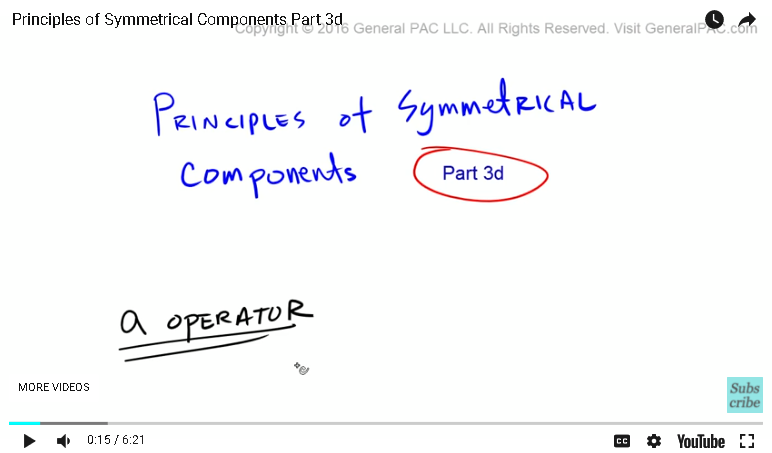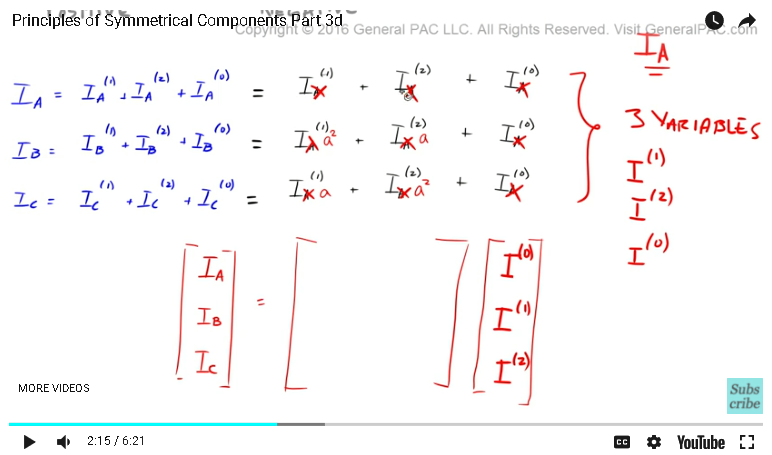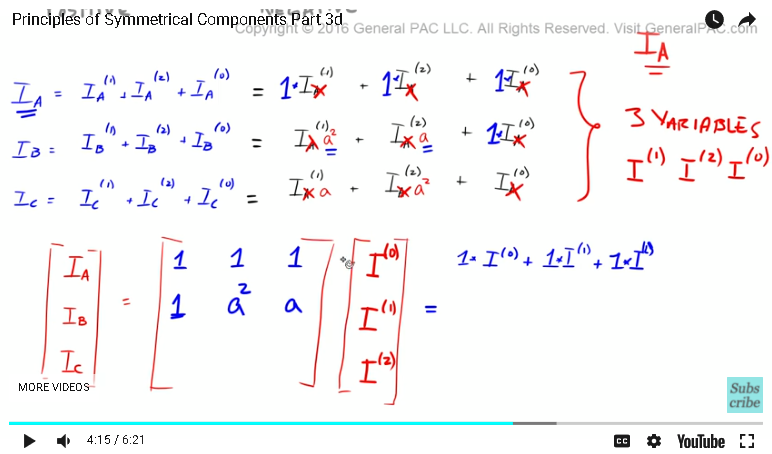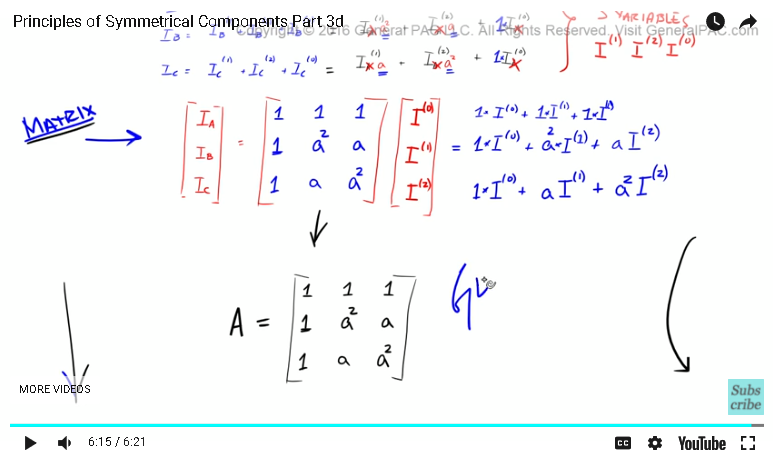### Resources Section

Web Application for Symmetrical Componets: http://personal.strath.ac.uk/steven.m.blair/seq/

Do you have a question? Click on the "Questions & Answers forum" and ask away!

Principles of symmetrical components. Okay, so in the previous tutorials, we've been talking about these a operators, and some of the benefits of the a operators from the symmetrical components point of view. In this video tutorial, we'll continue this discussion.Okay, so here's the cleaned up version of what we were looking at in the previous video tutorials. In this tutorial, we're going to represent these phasors in matrix form to get a better understanding of what that might look like. First of all, we're going to represent IA, IB, and IC like that. Then, we're going to say that we simply have one column and three rows of this matrix, okay? Now, that is equal to some column here effectively multiplied by some column there. We need to fill in this gap.

First, let's place our three variables in this column here. But before we do that, it's important to note that when we get to this point here, we all know that we're using IA, we're using IA as our reference variables. Because we already know that we're using IA for our reference variable, we don't necessarily have to show this anymore, because it's already assumed. We already know we're working with IA, we already know that IA is our reference variable. Okay, so with that given said, our three variables are this, I positive sequence, I negative sequence, and I zero sequence. We're going to put our three variables in this column here, but we will start with I zero sequence first. You will see why in just a little while. Then, we'll place I positive sequence right underneath, and then I negative sequence underneath that.

Now, let's represent these equation in this matrix. We've taken care of the variables that we're going to use, right? Now we have to fill in all the other stuff. For IA, we know that IA, which is this guy right here, that's going to equal just the positive sequence plus the negative sequence plus the zero sequence. But what this doesn't show is that if we just multiply everything by one, we could represent this in here. We'll start with zero sequence first. We'll say that we have one there, which represents zero sequence, and then we have one there, which represents positive sequence, and then we'll have one there which represents negative sequence, so IA is equal to one times zero sequence component. We're talking about one times zero sequence component, plus one times positive sequence component, plus one times negative sequence component. We're going to move this in the middle so that we have more room to work with. We've taken care of this row.Now, let's represent IB. IB, that's going to equal, let's start with the zero sequence component first. Zero sequence component is right there, so we just have one times zero sequence component So that is equal to one. Now, we have to represent positive sequence component, and this was positive sequence component for IB, but remember, now we have to take care of this a operator. So instead of one anymore, now we have to multiply a superscript two operator, which means that this becomes a superscript two, which represents positive sequence component. Then our negative sequence component, which is this guy right here, that is represented by just the a operator, so that goes right there. So IB is equal to one times zero sequence component, so one times zero sequence component plus a subscript two times the positive sequence component. I have a here, and then multiply that by the positive sequence component, plus a times the negative sequence component, so plus a times the negative sequence component. All right?IC with respect to the zero sequence component just has one right there. Then for the positive sequence component, it has just the a operator, so the a goes there, and then for the negative sequence component, the negative sequence component goes right there, and then we have a superscript two right there. So a superscript two. Which means that IC is equal to one times the zero sequence component, plus a times positive sequence component, plus a superscript two times negative sequence component. So a superscript two times negative sequence component. And there you have it.

What we now get is that this right here is the matrix form representing our symmetrical component. This is a very, very common representation in many books and many papers, but this is essentially how the symmetrical components are derived in this fashion. A lot of the times, this right here, this is represented by A is equal to this matrix, so I'm going to put that there. We're going to stop this video here.

Now, if you haven't subscribed already, please go ahead and click on the bottom right corner of your screen, and subscribe to this channel. If you have any questions, there's going to be a link to the forum on the bottom of this video. Ask away on that particular forum. Visit GeneralPac.com for more video tutorials similar to this one. Thank you.#### Greetings from the GeneralPAC Team!

We make high-quality Power Systems Video Tutorials on complex topics that are free and open to everyone!  Thank you so much for supporting us through Patreon so can continue doing good and valuable work.

What is Patreon and why do we use it?

Patreon is a fantastic portal that allows our fans and community to make monthly contribution (like Netflix subscription) so we can continue creating high-quality power systems video tutorials. In return, you get access to incredible perks like voting on future topics, getting your questions answered, access to VIP Q/A webinars with the creators of GeneralPAC, and much more! We THANK YOU for supporting us

Why do we need your support?

An incredible amount of time and effort is needed to develop high-quality video tutorials. Each video (Part 1 for example) takes approximately 10 hours to complete which includes learning the concept ourselves, brainstorming creative ways to teach and explain the concepts, writing the script, audio recording, video recording, and editing. It's no wonder why Hundreds-of-Thousands of people have watched, liked, subscribed, and left positive comments on Youtube channel. Your support truly makes all the difference.